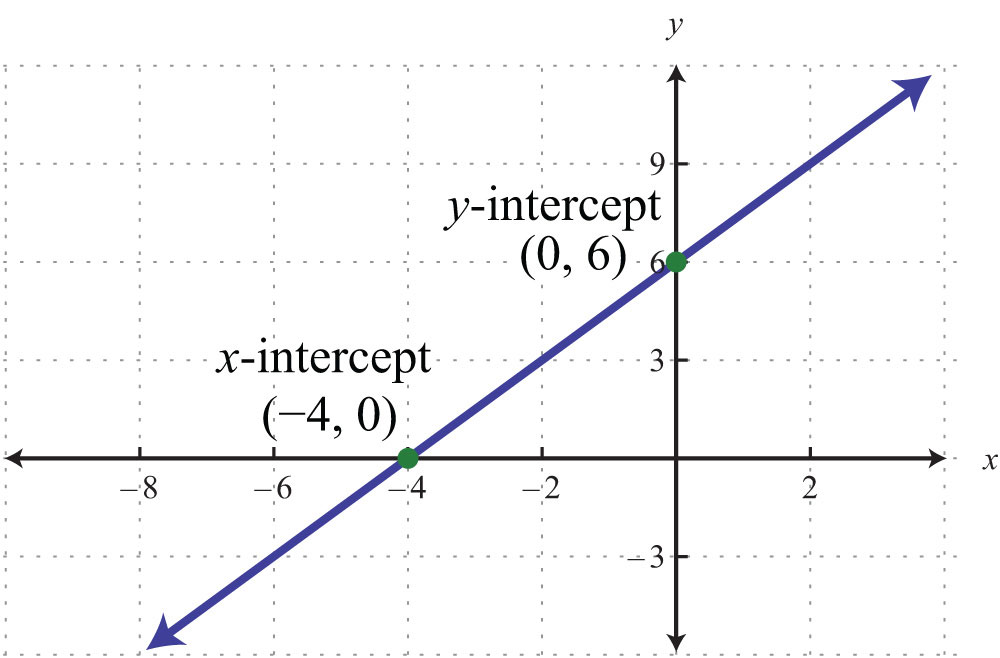# eQuizShow

## Graphing Linear Equations

### Slippery Slope

Question: A line with a slope of zero is what type of line, and has what type of equation?
Equation will be y=some number (no x)

Question: A line with an undefined slope is what type of line and has what type of equation?
Equation will be x = some number (no y)

Question: What kind of slope does the following graph have?Question: What type of slope will a line with the equation y = 3 have?

Question: Describe two possible ways to use the slope -3/4 to graph a point on a line.
Answer: You could use -3/4 and go 3 down and 4 to the right
OR
You could use 3/-4 and go 3 up and 4 to the left

### Intercept Junction

Question: In the slope intercept form y = mx + b the b stands for the ____________________.

Question: A y-intercept is the point where the graph crosses the _______________
An x-intercept is the point where the graph crosses the _____________
x axis

Question: Find the x and y intercepts for the following equation:

5x - 5y = 10
Answer: x-intercept = 2 or (2,0)

y-intercept = -2 or (0, -2)

Question: What are the coordinates of the x and y intercepts of this graph?y-intercept (0, 6)

Question: What are the x and y intercepts for the following equation?
7x - 3y = 12
Answer: x-intercept = 12/7 or 1 5/7 or (12/7, 0)
y-intercept = -4 or (0, -4)

### What's My Function?

Question: Find f(x) if x = 5 for the following function.

f(x) = 10x - 5

Question: If f(x) = 10 what is x for the following function?

f(x) = 3x - 2

Question: Find f(x) if x = 6 for the following function.
f(x) = 1/2x - 5

Question: If f(x) = 10 what is x for the following function?
f(x) = 1/2x - 2

Question: What is the function for this graph?Answer: f(x) = 3/2x + 6

### Why Solve For Y

Question: When an equation is in this format y = mx + b, we say it is in ________________ form.

Question: Solve this equation for y:

4x + y = 12
Answer: y = 12 - 4x or y = -4x + 12

Question: Solve for y.

2x - 2y = 12
Answer: y = -6 + x or y = x - 6

Question: Solve the following equation for y,

2y + 5x = 13
Answer: y = 13/2 - 5/2x OR y = -5/2x + 13/2

Question: Does the following equation show direct variation? If yes, what is the constant of variation?

2x - 4y = 0
Answer: Yes, the constant of variation is 1/2

### Graph It

Question: Graph this equation y = x
Answer:Question: Describe how to use a slope of 2/3 to graph a point on a line.
Answer: Move up 2 and to the right 3 to get from one point to the next.

Question: What is the y-intercept and the slope for the following equation.

2y + 9x = 18